MORE IN Circuits and Transmission Lines
MU Electronics and Telecom Engineering (Semester 3)
Circuits and Transmission Lines
December 2014
Total marks: --
Total time: --
INSTRUCTIONS
(1) Assume appropriate data and state your reasons
(2) Marks are given to the right of every question
(3) Draw neat diagrams wherever necessary

1 (a) Prove that F(s)=(s+?) where ? is a non-zero positive integers, is Hurwitz. Hence show that the polynomial P(s)=(s+6)2(s+4)6 is also Hurwitz.
5 M
1 (b) State and prove initial Value Theorem.
5 M
1 (c) Determine the ABCD parameters of the netwrork shown in fig. 1(c) considering the network as a cascade of two sub-networks.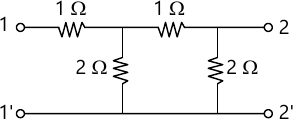5 M
1 (d) Find Foster I and II, and Cauer I and II circuits for the driving point admittance $Y(s)= \dfrac {s^2+1}{s}$ Comment on your result.
5 M

2 (a) Find the value of the variable resistance R in the circuit shown in Fig. 2(a) such that the power delivered to the load resistance Ri=2 ohm is maximum. Evaluate the maximum value of the power.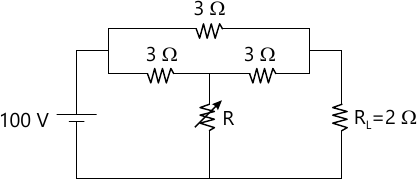10 M
2 (b) Two identical 2-port networks are connected in cascade. If the y-parameters of each network are y11=-y12=-y21-y22=10 mho determine the y-parameters of the composite network.
5 M
2 (c) In the circuit shown in Fig. 2(c) find Vx.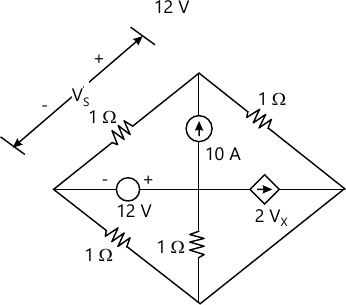5 M

3 (a) Test if $Z(s)=\dfrac {(s+1) (s+9)}{s(s+4)}$ represents a diving point impedance of an RL, RC or LC circuit. Sketch Z(σ) or Z(ω) curves whichever is applicable. Fost Foster II canonical circuit for the function.
10 M
3 (b) State and prove Final Value Theorem.
5 M
3 (c) The parameters of a transmission line are: 0.25 ? mho/km, 6&Omega/km, 2.22 mH/km, 005 ?F/km. Find the characteristics impedance Z0 and propagation constant ? at 1 Khz.
5 M

4 (a) Derive an expression for current I in the circuit shown in Fig 4(a) under the condition ?2 LC=0.5, where ? is the radian frequency of the ac voltage V. Hence show that |I| is independent of the impedance Zx.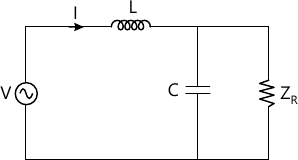10 M
4 (b) Test if $F(s) = \dfrac {s^3+10s^2+27s+18}{(s+1)(s+3)(s+5)}$ is a Positive Real Function. Realize the function as driving point impedance in Foster I form.
5 M
4 (c) Determine V1 for the network shown in Fig. 4(c) when L=CR2 and V1 is a pulse of height 10V and width 1 S.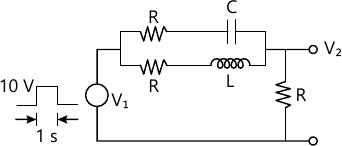5 M

5 (a) A unit step voltage is applied to a circuit consisting of only passive elements (each has numerical value I). The current supplied by the source is exponentially decreasing as shown in Fig. 5(a). Find the circuit with the minimum number of elements possible.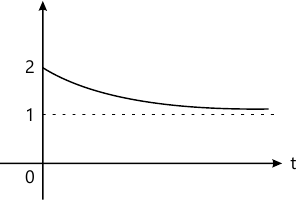10 M
5 (b) Define and give the significance of the following:
i) Characteristics Impedance
ii) Propagation constant
iii) Reflection coefficient
5 M
5 (c) State the conditions for a reciprocal 2-port network in terms of y-parameters and then convert it in terms of ABCD parameters.
5 M

6 (a) Write a brief note on Smith chart under the following heads.
i) VSWR circles
ii) Characteristics
iii) Applications.
A lossless 75? transmission line is terminated by an impedance of 150+j150?. Using Smith chart determine the following.
i) VSWR
ii) reflection coefficient
10 M
6 (b) Find Thevenin resistance of the circuit shown in Fig. 6(b) across the terminals AB.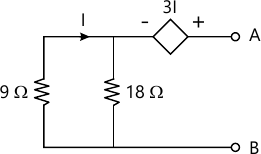5 M
6 (c) Determine the Laplace transform of v1(t) shown in Fig. 6(c)(i). Hence find the Laplace transform of v2(t) shown in Fig. 6(c)(ii).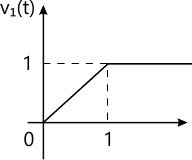Fig. 6(c)(i)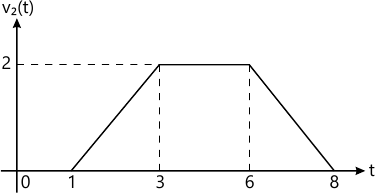Fig 6(c)(ii)

5 M

More question papers from Circuits and Transmission Lines